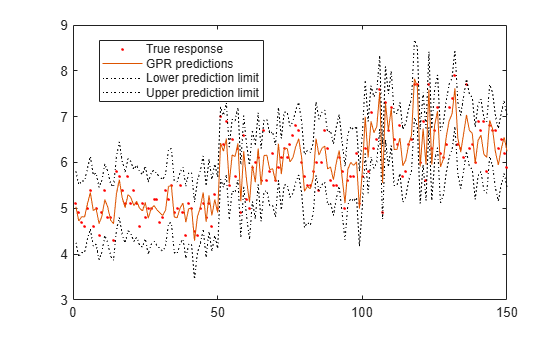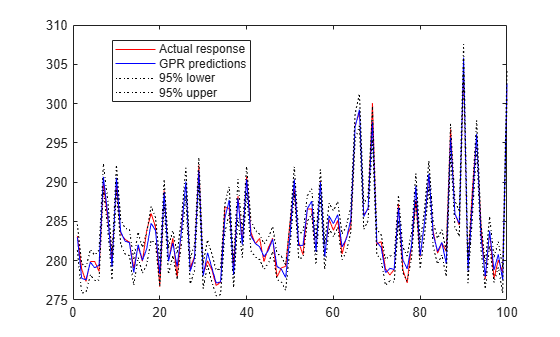# predict

Predict response of Gaussian process regression model

## Syntax

```ypred = predict(gprMdl,Xnew) [ypred,ysd,yint] = predict(gprMdl,Xnew) [ypred,ysd,yint] = predict(gprMdl,Xnew,'Alpha',alpha) ```

## Description

`ypred = predict(gprMdl,Xnew)` returns the predicted responses `ypred` for the Gaussian process regression (GPR) model `gprMdl` and the predictor values in `Xnew`.

```[ypred,ysd,yint] = predict(gprMdl,Xnew)``` also returns the standard deviations `ysd` and 95% prediction intervals `yint` of the response variable, evaluated at each observation in `Xnew` using the trained GPR model.

```[ypred,ysd,yint] = predict(gprMdl,Xnew,'Alpha',alpha)``` specifies the significance level for the confidence level of the prediction intervals `yint`. The confidence level of `yint` is equal to `100(1 – Alpha)%`.

## Input Arguments

expand all

Gaussian process regression model, specified as a `RegressionGP` (full) or `CompactRegressionGP` (compact) object.

New values for the predictors that `fitrgp` uses in training the GPR model, specified as a `table` or an m-by-d matrix. m is the number of observations and d is the number of predictor variables in the training data.

If you trained `gprMdl` on a `table`, then `Xnew` must be a `table` that contains all the predictor variables used to train `gprMdl`.

If you trained `gprMdl` on a matrix, then `Xnew` must be a numeric matrix with d columns.

Data Types: `single` | `double` | `table`

Significance level for the confidence level of the prediction intervals `yint`, specified as a numeric scalar in the range `[0,1]`. The confidence level of `yint` is equal to `100(1 – Alpha)%`.

Example: `'Alpha',0.01` specifies to return 99% prediction intervals.

Data Types: `single` | `double`

## Output Arguments

expand all

Predicted responses, returned as a column vector of length n, where n is the number of observations in the predictor data `Xnew`.

Standard deviations of the response variable, evaluated at each observation in the predictor data `Xnew`, returned as a column vector of length n, where n is the number of observations in `Xnew`. The `i`th element `ysd(i)` contains the standard deviation of the `i`th response for the `i`th observation `Xnew(i,:)`, estimated using the trained GPR model `gprMdl`.

Prediction intervals of the response variable, evaluated at each observation in the predictor data `Xnew`, returned as an n-by-2 matrix, where n is the number of observations in `Xnew`. The `i`th row `yint(i,:)` contains the `100(1 – Alpha)%` prediction interval of the `i`th response for the `i`th observation `Xnew(i,:)`. The `Alpha` value is the probability that the prediction interval does not contain the true response value for `Xnew(i,:)`. The first column of `yint` contains the lower limits of the prediction intervals, and the second column contains the upper limits.

## Examples

expand all

Generate the sample data.

```n = 10000; rng(1) % For reproducibility x = linspace(0.5,2.5,n)'; y = sin(10*pi.*x) ./ (2.*x)+(x-1).^4 + 1.5*rand(n,1);```

Fit a GPR model using the Matern 3/2 kernel function with separate length scale for each predictor and an active set size of 100. Use the subset of regressors approximation method for parameter estimation and fully independent conditional method for prediction.

```gprMdl = fitrgp(x,y,'KernelFunction','ardmatern32', ... 'ActiveSetSize',100,'FitMethod','sr','PredictMethod','fic');```

Compute the predictions.

`[ypred,~,yci] = predict(gprMdl,x);`

Plot the data along with the predictions and prediction intervals.

```plot(x,y,'r.') hold on plot(x,ypred,'b-') plot(x,yci(:,1),'k--') plot(x,yci(:,2),'k--') xlabel('x') ylabel('y') legend('True responses','GPR predictions', ... 'Prediction interval limits','Location','best')```Load the sample data and store in a `table`.

```load fisheriris tbl = table(meas(:,1),meas(:,2),meas(:,3),meas(:,4),species,... 'VariableNames',{'meas1','meas2','meas3','meas4','species'});```

Fit a GPR model using the first measurement as the response and the other variables as the predictors.

`mdl = fitrgp(tbl,'meas1');`

Compute the predictions and the 99% confidence intervals.

`[ypred,~,yci] = predict(mdl,tbl,'Alpha',0.01);`

Plot the true response and the predictions along with the prediction intervals.

```figure(); plot(mdl.Y,'r.'); hold on; plot(ypred); plot(yci(:,1),'k:'); plot(yci(:,2),'k:'); legend('True response','GPR predictions',... 'Lower prediction limit','Upper prediction limit',... 'Location','Best');````load('gprdata.mat');`

The data contains training and test data. There are 500 observations in training data and 100 observations in test data. The data has 6 predictor variables. This is simulated data.

Fit a GPR model using the squared exponential kernel function with a separate length scale for each predictor. Standardize predictors in the training data. Use the exact fitting and prediction methods.

```gprMdl = fitrgp(Xtrain,ytrain,'Basis','constant',... 'FitMethod','exact','PredictMethod','exact',... 'KernelFunction','ardsquaredexponential','Standardize',1);```

Predict the responses for test data.

`[ytestpred,~,ytestci] = predict(gprMdl,Xtest);`

Plot the test response along with the predictions.

```figure; plot(ytest,'r'); hold on; plot(ytestpred,'b'); plot(ytestci(:,1),'k:'); plot(ytestci(:,2),'k:'); legend('Actual response','GPR predictions',... '95% lower','95% upper','Location','Best'); hold off```## Tips

• You can choose the prediction method while training the GPR model using the `PredictMethod` name-value pair argument in `fitrgp`. The default prediction method is `'exact'` for n ≤ 10000, where n is the number of observations in the training data, and `'bcd'` (block coordinate descent), otherwise.

• Computation of standard deviations, `ysd`, and prediction intervals, `yint`, is not supported when `PredictMethod` is `'bcd'`.

• If `gprMdl` is a `CompactRegressionGP` object, you cannot compute standard deviations, `ysd`, or prediction intervals, `yint`, for `PredictMethod` equal to `'sr'` or `'fic'`. To compute `ysd` and `yint` for `PredictMethod` equal to `'sr'` or `'fic'`, use the full regression (`RegressionGP`) object.

## Alternatives

You can use `resubPredict` to compute the predicted responses for the trained GPR model at the observations in the training data.

## Version History

Introduced in R2015b